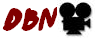© | << < ? > >> | Dror Bar-Natan: Talks:

# Some Feynman Diagrams in Algebra

### UCLA Topology Seminar, November 1, 2019.

Abstract. I will explain how the computation of compositions of maps of a certain natural class, from one polynomial ring into another, naturally leads to a certain composition operation of quadratics and to Feynman diagrams. I will also explain, with very little detail, how this is used in the construction of some very well-behaved poly-time computable knot polynomials.

Joint work with Roland van der Veen.

Handout: FDA.html, FDA.pdf, FDA.png.Talk Video.

Links: Ov akt hm sl2

Sources: pensieve.

## Everything around $sl_{2+}^\epsilon$ is DoPeGDO. So what?

### UCLA Topology Seminar, November 1, 2019.

Abstract. I'll explain what "everything around" means: classical and quantum $m$, $\Delta$, $S$, $tr$, $R$, $C$, and $\theta$, as well as $P$, $\Phi$, $J$, ${\mathbb D}$, and more, and all of their compositions. What DoPeGDO means: the category of Docile Perturbed Gaussian Differential Operators. And what $sl_{2+}^\epsilon$ means: a solvable approximation of the semi-simple Lie algebra $sl_2$.

Knot theorists should rejoice because all this leads to very powerful and well-behaved poly-time-computable knot invariants. Quantum algebraists should rejoice because it's a realistic playground for testing complicated equations and theories.

This is joint work with Roland van der Veen and continues work by Rozansky and Overbay.

Handout: DoPeGDO.html, DoPeGDO.pdf, DoPeGDO.png.See videos at DaNang-1905 and at CRM-1907.

Sources: pensieve.